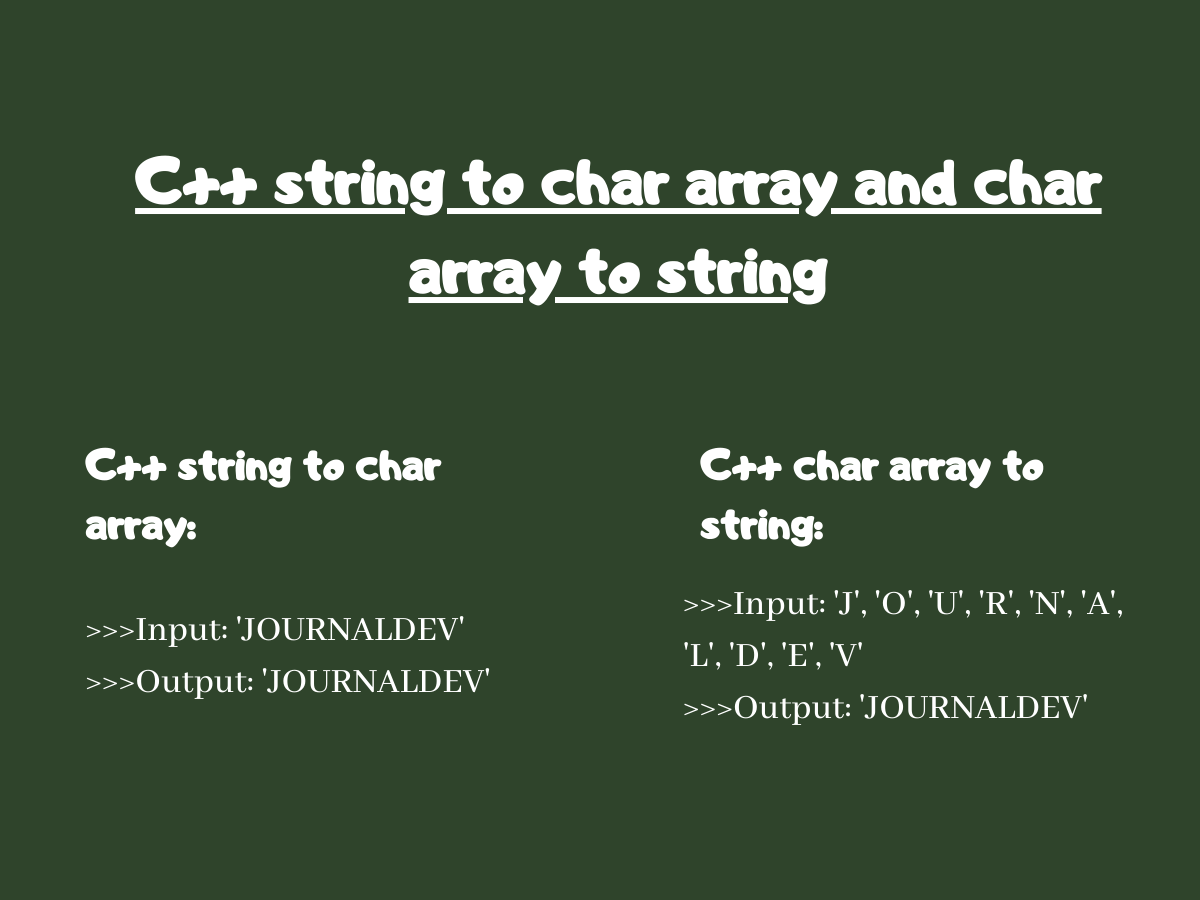# Convert String to Char Array and Char Array to String in C++

Published on August 3, 2022By Safa MulaniWhile we believe that this content benefits our community, we have not yet thoroughly reviewed it. If you have any suggestions for improvements, please let us know by clicking the “report an issue“ button at the bottom of the tutorial.

In this article, we will be focusing on the different ways to convert String to char array and char array to String in C++. While dealing with String data, we may need to convert the string data items to character array and vice-versa. This tutorial will help you solve exactly that.

## Convert String to Char Array in C++

C++ provides us with the following techniques to convert a string to char array:

• Using c_str() and strcpy() function
• Using a for loop

### 1. The c_str() and strcpy() function in C++

C++ `c_str()` function along with C++ String `strcpy()` function can be used to convert a string to char array easily.

The c_str() method represents the sequence of characters in an array of string followed by a null character (‘\0’). It returns a null pointer to the string.

Syntax:

``````string-name.c_str();
``````
• At first, we use c_str() method to get all the characters of the string along with a terminating null character.
• Further, we declare an empty array of type char to store the result i.e. result of the conversion of string to char array.
• Finally, we use strcpy() method to copy the character sequence generated by the c_str() method to the empty char array.

Example:

``````#include <bits/stdc++.h>
using namespace std;

int main()
{

string str = "";
cout<<"Enter the string:\n";

cin>>str;

char arr[str.length() + 1];

strcpy(arr, str.c_str());
cout<<"String to char array conversion:\n";
for (int i = 0; i < str.length(); i++)
cout << arr[i];

return 0;
}

``````

Output:

``````Enter the string:
JournalDev
String to char array conversion:
JournalDev
``````

### 2. String to Char Array Conversion in C++ Using for Loop in

For the conversion of char array to a string, we can use C++ for loops with ease.

• Initially, we create an empty array of type char
• After this, we iterate through the input string
• While iterating, we store the characters into the char array

Example:

``````#include <bits/stdc++.h>
using namespace std;

int main()
{

string str = "";
cout<<"Enter the string:\n";

cin>>str;

char arr[str.length() + 1];
cout<<"String to char array conversion:\n";
for (int x = 0; x < sizeof(arr); x++) {
arr[x] = str[x];
cout << arr[x];
}

return 0;
}

``````

Output:

``````Enter the string:
JournalDev
String to char array conversion:
JournalDev
``````

## Convert Char Array to String in C++

The mentioned techniques can be used to convert a char array to string in C++:

• The ‘+’ operator
• C++ inbuilt-constructor

### 1. C++ ‘+’ operator

C++ provides us with `'+' operator` to concatenate or add data items to a variable.

• We create a new empty string to store the result.
• Taking it ahead, we use a for loop to traverse through the input char array.
• In the process of traversing through the array, we use ‘+’ operator to concatenate the characters to the string.

Example:

``````#include <bits/stdc++.h>
using namespace std;

int main()
{
char arr[] = { 'J', 'O', 'U', 'R', 'N', 'A', 'L', 'D', 'E', 'V' };

int size_arr = sizeof(arr) / sizeof(char);
string str = "";
for (int x = 0; x < size_arr; x++) {
str = str + arr[x];
}
cout<<"Converted char array to string:\n";
cout << str << endl;
return 0;
}

``````

Output:

``````Converted char array to string:
JOURNALDEV
``````

### 2. C++ overloaded ‘=’ operator

C++ has got the concept of overloading which makes an operator perform other operations apart from the basic or default operation.

• Initially, we create a new empty string.
• We use the overloaded `'=' operator` to store the data items character by character into the newly created empty string.

Example:

``````#include <bits/stdc++.h>
using namespace std;

int main()
{
char arr[] = { 'J', 'O', 'U', 'R', 'N', 'A', 'L', 'D', 'E', 'V' };

int size_arr = sizeof(arr) / sizeof(char);
string str = "";
str = arr;
cout<<"Converted char array to string:\n";
cout << str << endl;
return 0;
}

``````

Output:

``````Converted char array to string:
JOURNALDEV
``````

### 3.C++ String inbuilt constructor

In the context of conversion of char array to string, we can use C++ String Constructor for the same.

Syntax:

``````string string-name(char array-name);
``````

This constructor takes a sequence of characters terminated by a null character as an input parameter.

Note: This `constructor string string()` can be used only at the time of string declaration throughout the program.

Example:

``````#include <bits/stdc++.h>
using namespace std;

int main()
{
char arr[] = { 'J', 'O', 'U', 'R', 'N', 'A', 'L', 'D', 'E', 'V' };
int size_arr = sizeof(arr) / sizeof(char);
string str(arr);
cout<<"Converted char array to string:\n";
cout <<str<< endl;
return 0;
}

``````

Output:

``````Converted char array to string:
JOURNALDEV
``````

## Conclusion

In this article, we have understood various techniques to convert string to char array and vice-versa in C++.

Click below to sign up and get \$200 of credit to try our products over 60 days!

### Popular Topics##### Hollie's Hub for Good

Working on improving health and education, reducing inequality, and spurring economic growth? We’d like to help.##### Become a contributor

You get paid; we donate to tech nonprofits.

##### Welcome to the developer cloud

DigitalOcean makes it simple to launch in the cloud and scale up as you grow – whether you’re running one virtual machine or ten thousand.© 2023 DigitalOcean, LLC.